# 信息是如何表示和存储的

## 信息存储

### 二进制与十六进制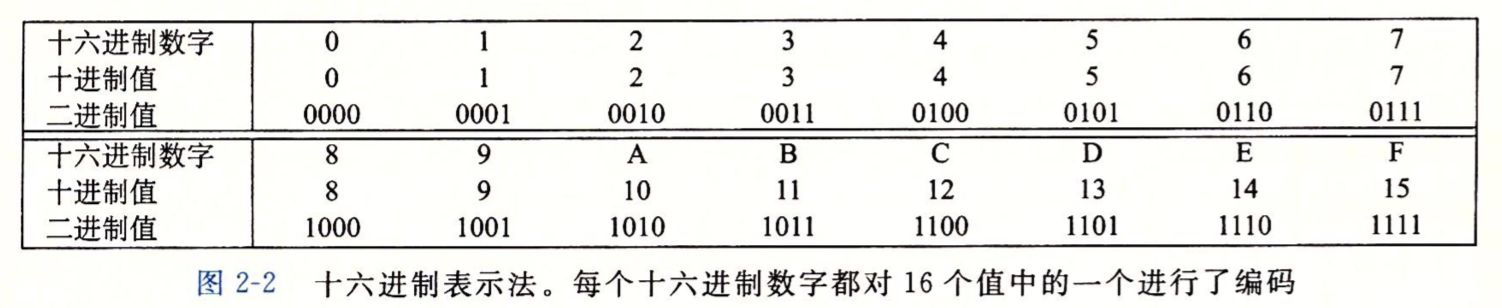1、十六进制与十进制转换：

2、十进制与十六进制转换：

3、二进制与十进制转换：

4、十进制与二进制转换：

w为数字长度，N为进制。

### 位运算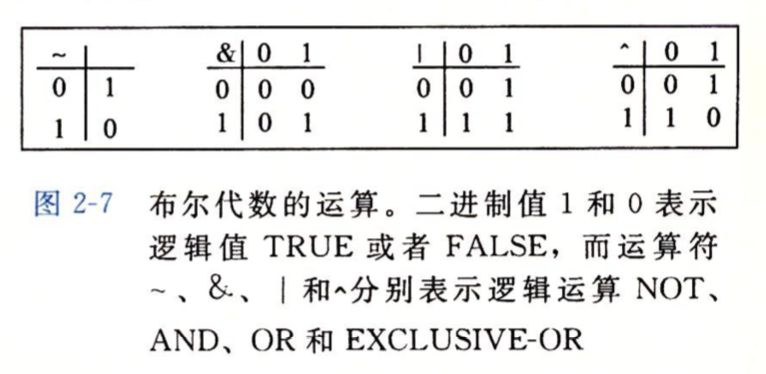• 与：全1为1
• 或：有1为1
• 异或：相异为1

### 移位运算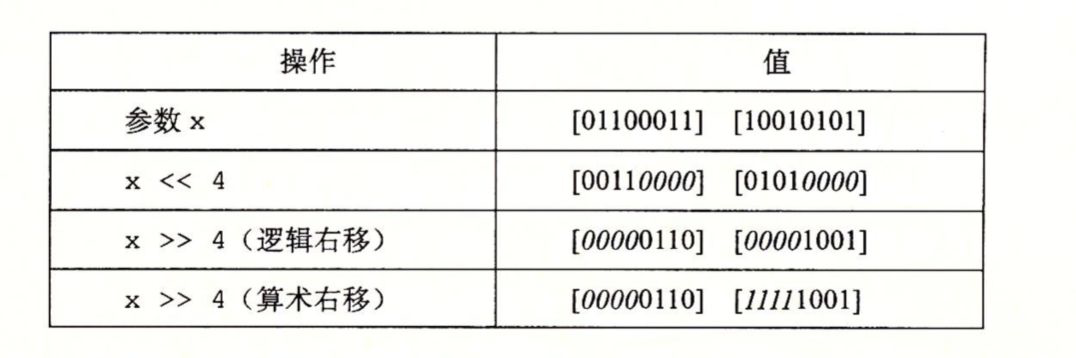## 整数表示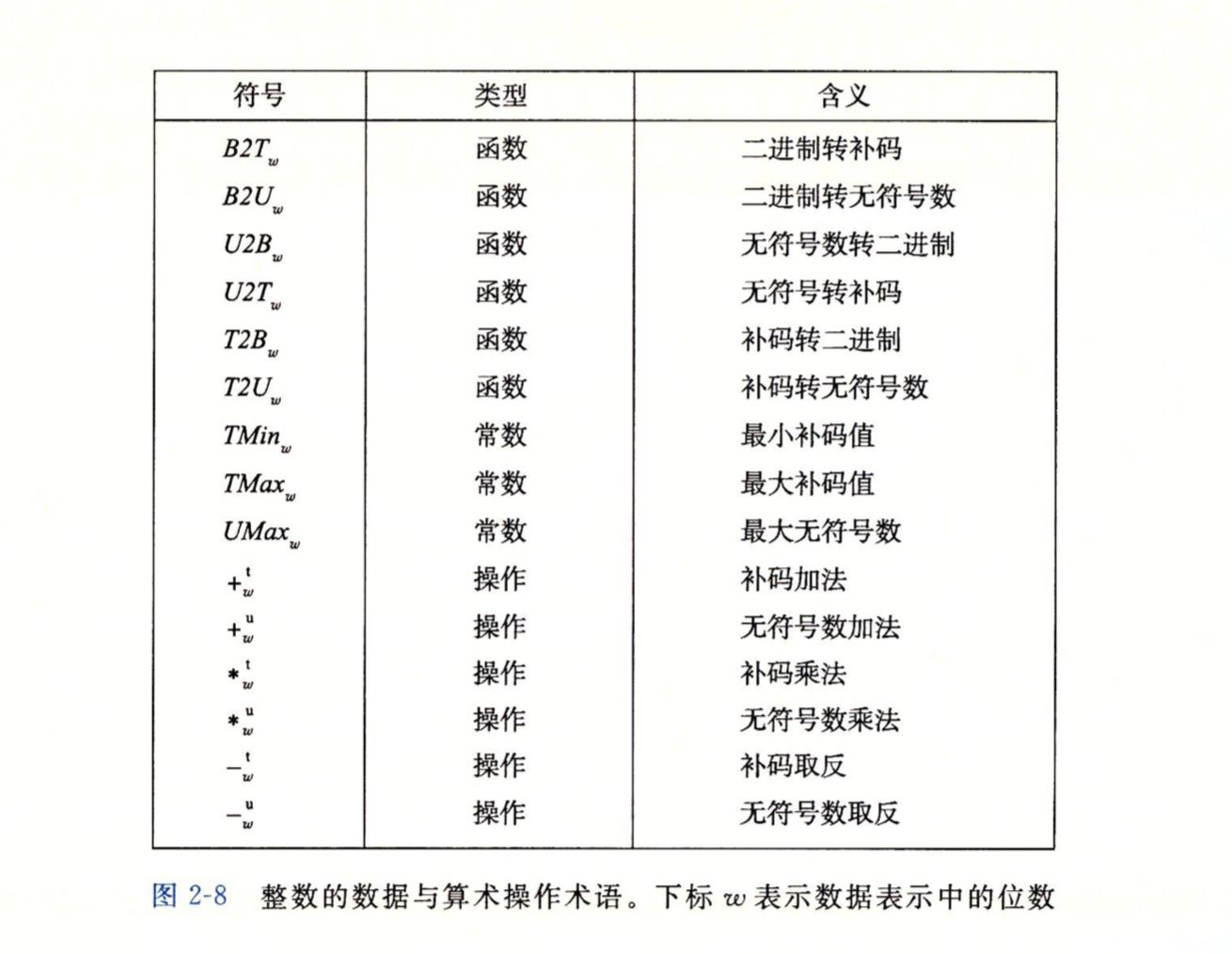### 整数数据类型

C 语言是支持多种整型数据类型的，下面我们看一下在 32 位机器和 64 位机器中，C 语言整型数据类型的取值范围：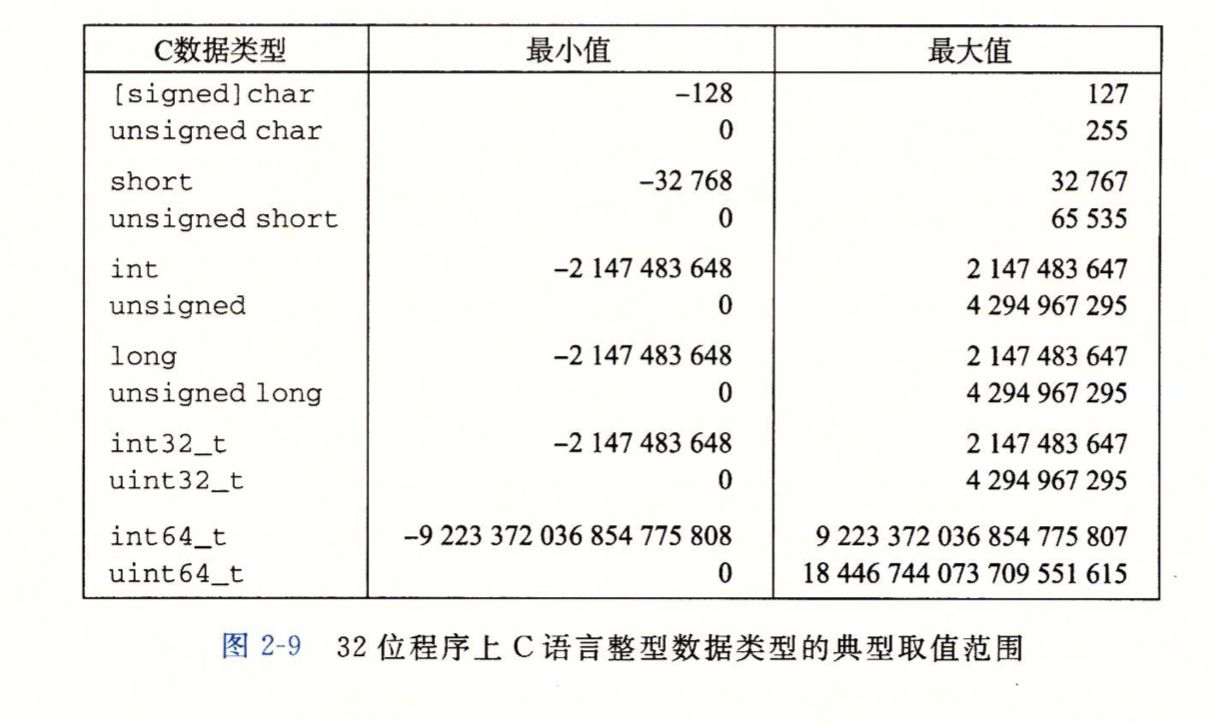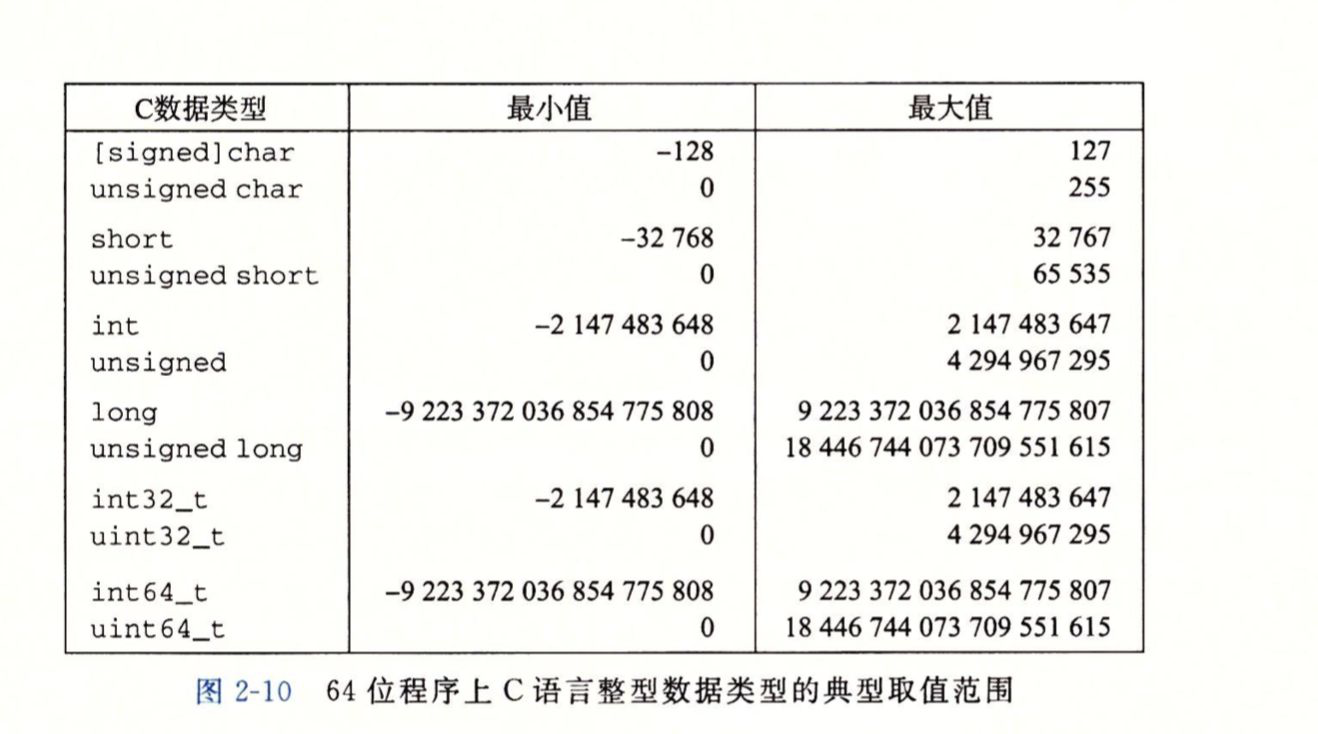• 数据类型分配的字节数会根据机器的字长和编译器有所不同，不同的大小所表示的范围是不同的。例如 long 类型。
• 负数的范围要比正数的范围大1。（为什么？）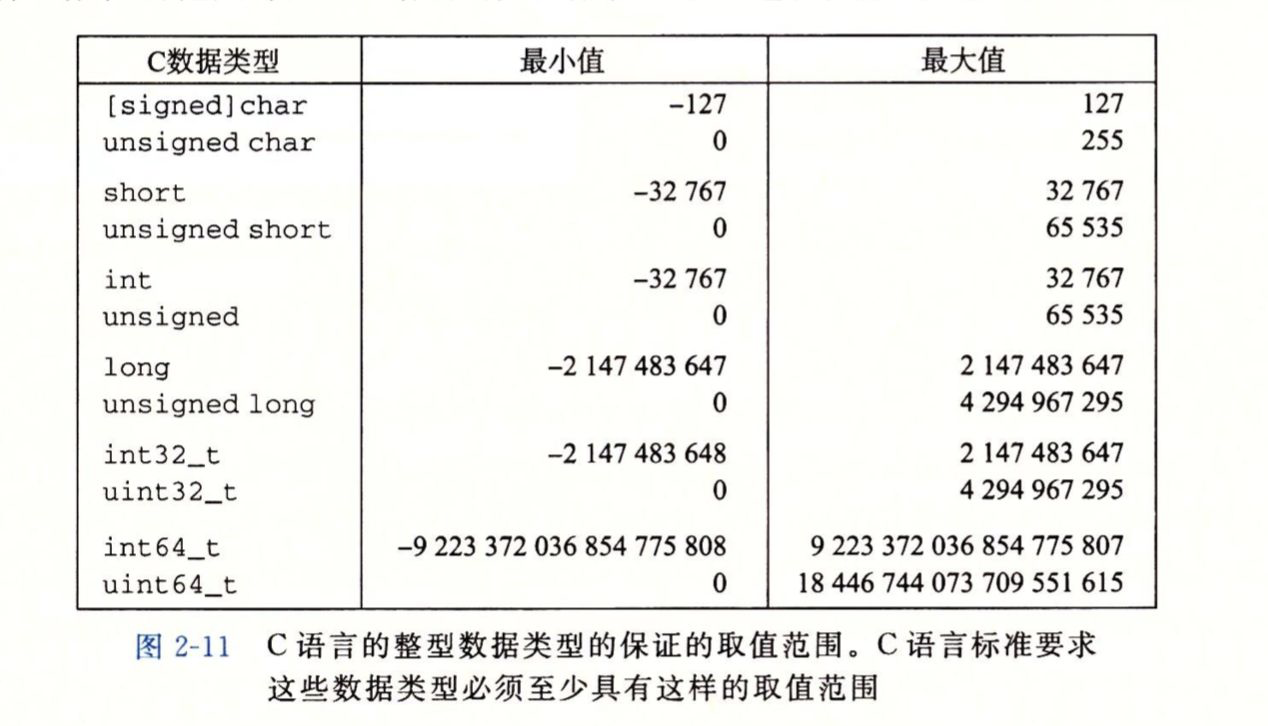### 无符号数编码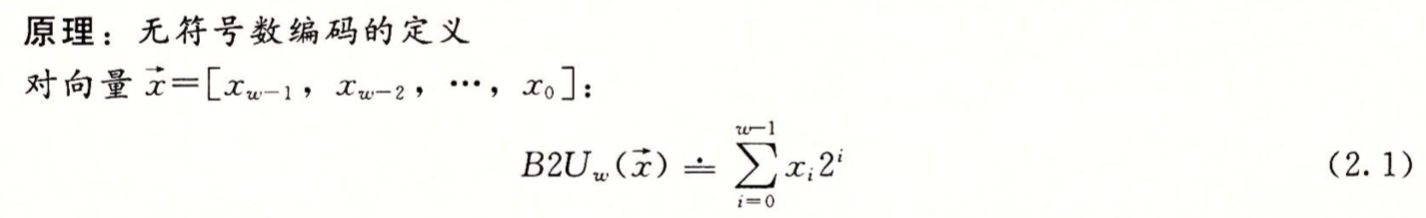w指的是一个w位的二进制数。下面是一个示例：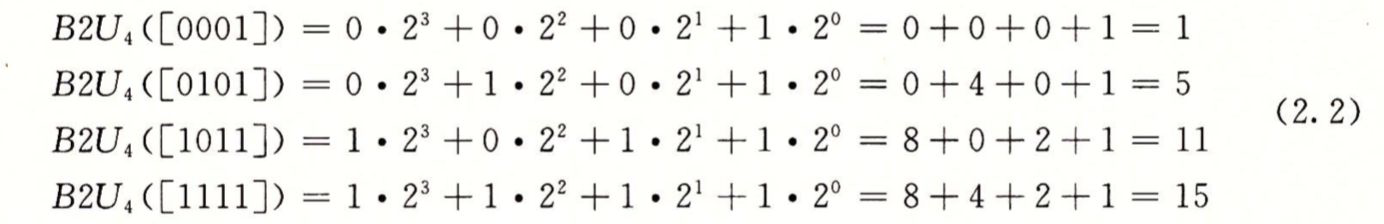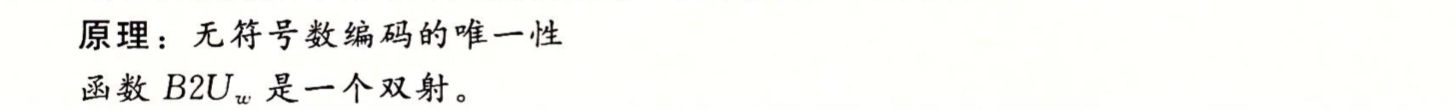### 补码编码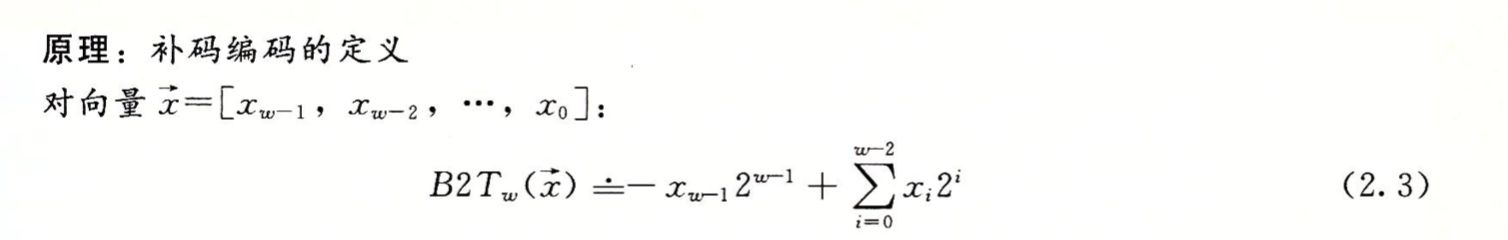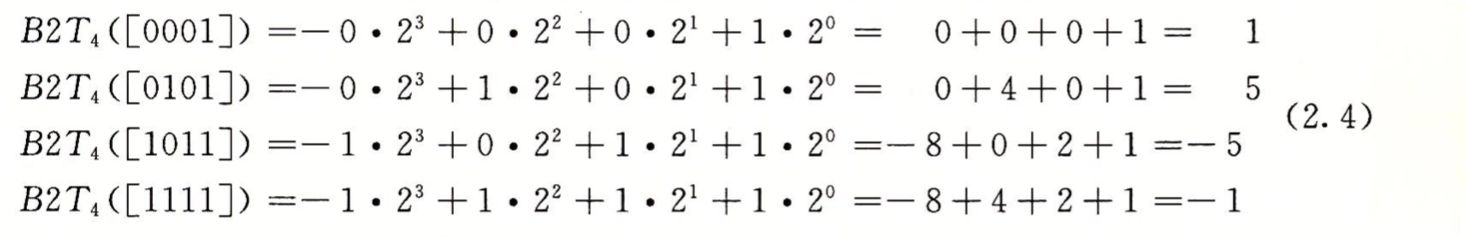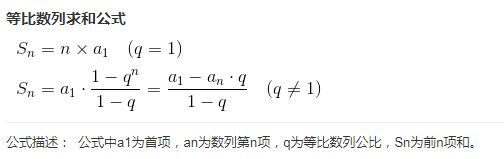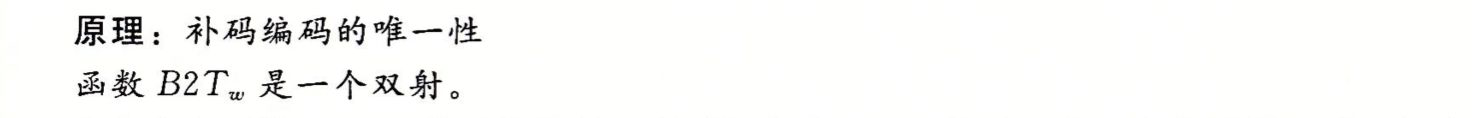### 无符号数转补码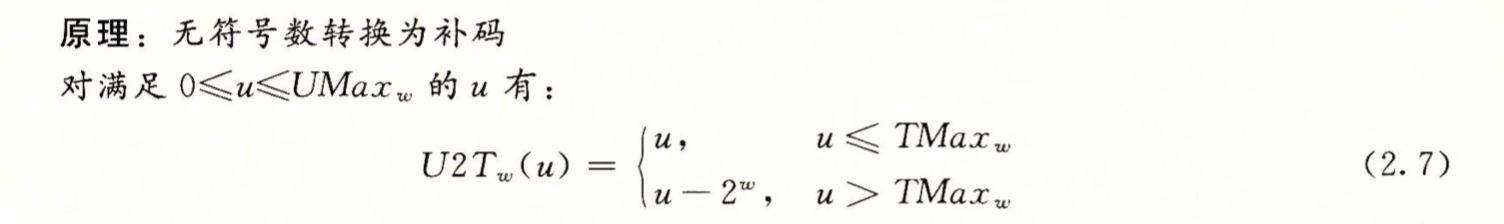w=8，u=255，$TMax_w = 2^7-1=127$，结果是 : $255 - 2^8 = -1$

w=8，u=126，$TMax_w = 2^7-1=127$，结果是 : 126。

C代码：

#include <stdio.h>

int main()
{
unsigned char u = 126;
char t = (char)u;
//%d把对应的整数按有符号十进制输出，%u把对应的整数按无符号十进制输出
printf("u=%u,u2t=%d\n",u,t);
return 0;
}


$gcc t1.c -o t1 && ./t1 u=126,u2t=126  ### 补码转无符号数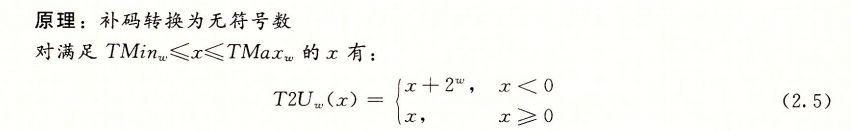示例： w=8，x=-1，结果是 : $-1 + 2^8 = 255$ w=8，x=126，结果是 : 126。 C代码： #include <stdio.h> int main() { char t = -1; unsigned char u = (unsigned char)t; printf("t=%d,t2u=%u\n",t,u); return 0; }  输出： $ gcc t1.c -o t1 && ./t1
t=-1,t2u=255


### 原码和反码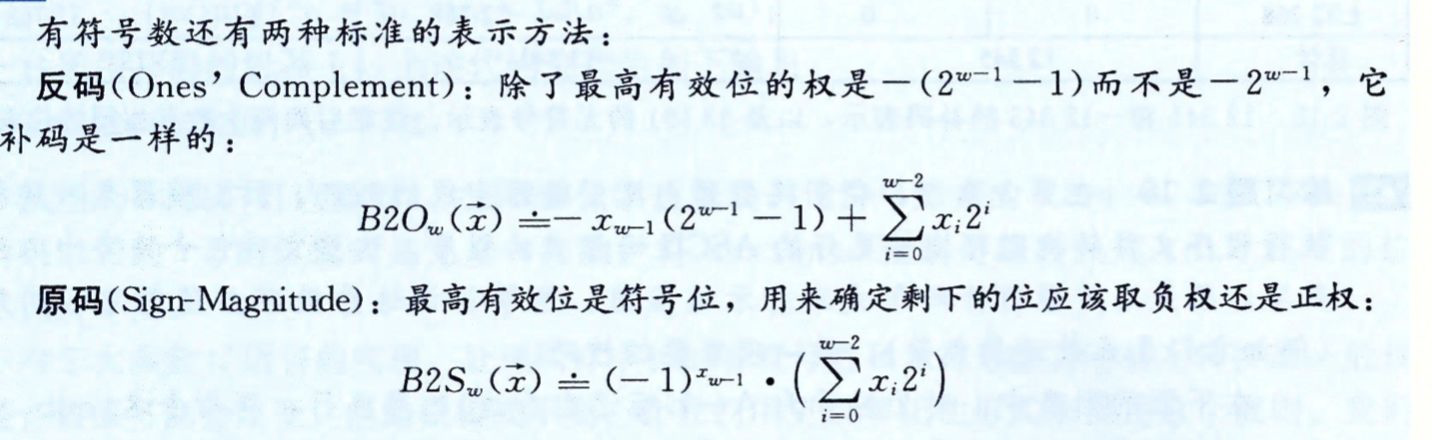1的原码为：00000001， 反码为：00000001， 补码为：00000001
-1的原码为：10000001，反码为：11111110，补码为：11111111
+0的原码为：00000000， 反码为：00000000， 补码为：00000000
-0的原码为：10000000，反码为：11111111，补码为：00000000
（反码加1产生了进位，变成100000000，最高位舍弃掉了）


### 扩展一个数字的位表示

1. 将一个无符号数转换为一个更大的数据类型，我们只需要简单的在二进制序列前面添加 0 即可。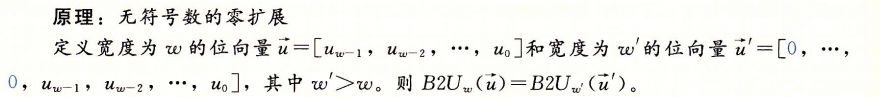1. 将一个补码数字转换为一个更大的数据类型，我们需要在开头添加符号位。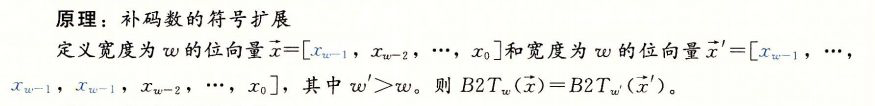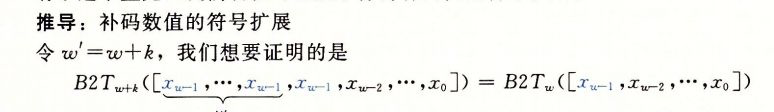$B2T_{w} = -x_{w-1}2^{w-1} + \sum^{w-2}_{i=0}x_i2^i$ 可得出 $B2T_{w+1}$ 展开后的值：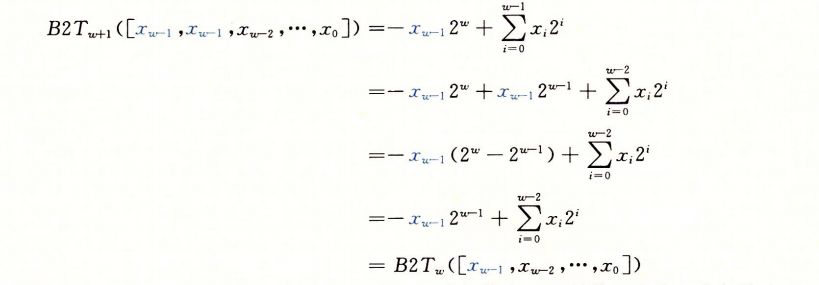$2^w - 2^{w-1} = 2^{w-1+1} - 2^{w-1} = 2^{w-1} * 2 - 2^{w-1} = 2^{w-1}$

w=4, 有符号二进制数 $1001_2 = -2^3 + 0 + 0 + 1 = -7$；

C代码：

#include <stdio.h>

int main()
{
char t = -7;
short int t2 = (short int)t;

printf("t=%d,t2=%d\n",t,t2);
return 0;
}


$gcc t1.c -o t1 && ./t1 t=-7,t2=-7  ### 截断数字 假设我们需要把一个数的位数减少，例如由 short int 变成 1字节的 char类型，这就需要使用截断原理了。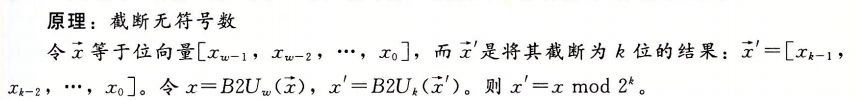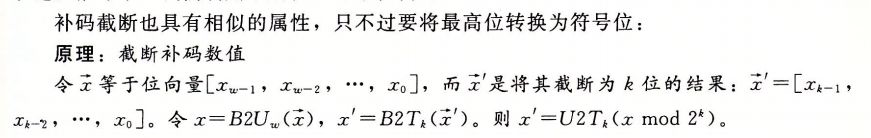示例： w=8, 有符号二进制数$11111001_2 = -7 $；截断4位，得到：$1001_2 = U2T_k( 9 mod 16 ) = U2T_k(9) = 9 - 2^4= -7 $，其中：$TMax_w = 2^3-1 = 7$附：## 整数运算 ### 无符号加法 原理：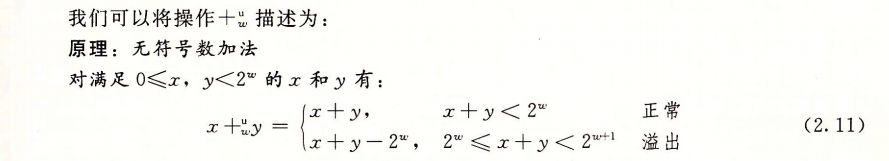注意：当 $2^w$ <= x+y <$2^{w+1}$，对 x + y 进行$2^w$的取模运算，与 x + y - $2^w$是等价的。 示例： #include <stdio.h> int main() { unsigned short int i = 65535; unsigned short int j = i+2; printf("%u\n",j); return 0; }  运行结果： $ gcc t1.c -o t1 && ./t1
1


### 补码加法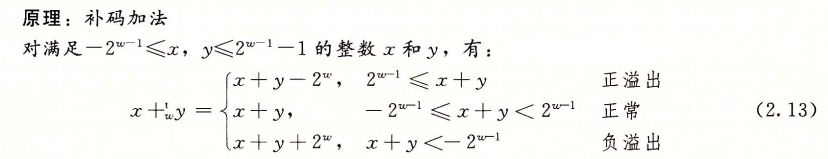#include <stdio.h>

int main()
{
short int i = -32768;
short int j = i-2;
printf("%u\n",j);
return 0;
}


### 二进制小数

1、二进制转为十进制：以小数点为界，整数位从最后一 位（从右向左）开始算，依次列为第0、1、2、3………n，然后将第n位的数（0或1）乘以2的n-1次方，然后相加即可得到整数位的十进制数；小数位则 从左向右开始算，依次列为第1、2、3……..n，然后将第n位的数（0或1）乘以2的-n次方，然后相加即可得到小数位的十进制数（按权相加法）。。

(10.101)2 = 1 * 2^1 + 0* 2^0 + 1*2^-1+ 0*2^-2+ 1*2^-3 = 2+0+0.5+0+0.125 = 2.625


2、十进制转为二进制：整数部分转换规则不变：不断用商除以2，直到商为0，再把得到的余数倒序就得到对应进制的数；小数部分不断乘以2，得到的数的整数部分按顺序保留，小数部分不为0则继续乘以2，最终小数部分由各整数部分组成的数字就是转化后二进制小数的值（正序）。

10 /2 = 5...0, 5 / 2 = 2...1, 2 /2 = 1...0, 1/2=0...1 所以整数部分的二进制是：1010
0.4 * 2 = 0.8, 整数部分是0，小数位不为0，继续；
0.8 * 2 = 1.6, 整数部分是1，
0.6*2 = 1.2 , 整数部分是1，
0.2*2 = 0.4 , 整数部分是0，
0.4*2 = 0.8, 整数部分是0，
...


(本文完)posted @ 2021-10-13 08:43  飞鸿影  阅读(3)  评论(0编辑  收藏  举报